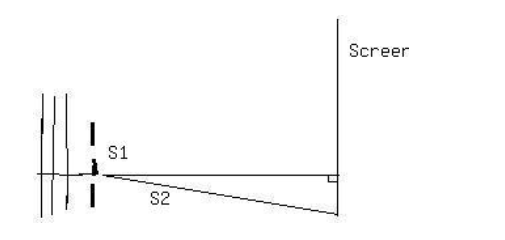Ask question

# Find the equation of the sphere centered at (-9, 3, 9) with radius 5. Give an equation which describes the intersection of this sphere with the plane z = 10.# Find the equation of the sphere centered at (-9, 3, 9) with radius 5. Give an equation which describes the intersection of this sphere with the plane z = 10.

Question
Solid Geometryasked 2021-03-02
Find the equation of the sphere centered at (-9, 3, 9) with radius 5. Give an equation which describes the intersection of this sphere with the plane z = 10.

## Answers (1)2021-03-03
Given: A circle with Center C = (-9,3,9) Radius r=5
General eqation for a sphere with center $$C=(x_0,y_0,z_0)$$ and radius r.
$$(x-x_0)^2+(y-y_0)^2+(z-z_0)^2=r^2$$
Replace $$x_0$$ with -9, $$y_0$$ with 3, $$z_0$$ with 9 and r with 5.
$$(x-(-9))^2+(y-3)^2+(z-9)^2-5^2$$
Simplify:
$$(x+9)^2+(y-3)^2+(z-9)^2=25$$
The intersection of the sphere with the plane z=10 can then be found by replacing z in the equation of the sphere by 10.
$$(x+9)^2+(y-3)^2+(10-9)^2=25$$
Simplify:
$$(x+9)^2+(y-3)^2+1=25$$
Subtract 1 from each side:
$$(x+9)^2+(y-3)^2=24$$
CONCLUSION
Equation sphere: $$(x+9)^2+(y-3)^2+(10-9)^2=25$$
Equation intersection with plane z=10: $$(x+9)^2+(y-3)^2=24$$

### Relevant Questionsasked 2021-02-18
Two hollow metal spheres are concentric with each other. Theinner sphere has a radius of 0.131 m and a potential of 89.6 V. Theradius of the outer sphere is 0.155 m and its potential is 78.3 V.If the region between the spheres is filled with Teflon, find theelectric energy contained in this space.asked 2021-05-04
The radius of a sphere increases at a rate of $$4m/\sec$$. Find the rate at which the surface area increases when the radius is 9m.
$$288\pi m^{2}/\sec$$
$$36\pi m^{2}/\sec$$
$$18\pi m^{2}/\sec$$
$$72\pi m^{2}/\sec$$
$$144\pi m^{2}/\sec$$asked 2021-04-25
The unstable nucleus uranium-236 can be regarded as auniformly charged sphere of charge Q=+92e and radius $$\displaystyle{R}={7.4}\times{10}^{{-{15}}}$$ m. In nuclear fission, this can divide into twosmaller nuclei, each of 1/2 the charge and 1/2 the voume of theoriginal uranium-236 nucleus. This is one of the reactionsthat occurred n the nuclear weapon that exploded over Hiroshima, Japan in August 1945.
A. Find the radii of the two "daughter" nuclei of charge+46e.
B. In a simple model for the fission process, immediatelyafter the uranium-236 nucleus has undergone fission the "daughter"nuclei are at rest and just touching. Calculate the kineticenergy that each of the "daughter" nuclei will have when they arevery far apart.
C. In this model the sum of the kinetic energies of the two"daughter" nuclei is the energy released by the fission of oneuranium-236 nucleus. Calculate the energy released by thefission of 10.0 kg of uranium-236. The atomic mass ofuranium-236 is 236 u, where 1 u = 1 atomic mass unit $$\displaystyle={1.66}\times{10}^{{-{27}}}$$ kg. Express your answer both in joules and in kilotonsof TNT (1 kiloton of TNT releases 4.18 x 10^12 J when itexplodes).asked 2020-12-28
Surface area Suppose that the radius rr and surface area $$S=4πr2S=4πr^{2}$$
of a sphere are differentiable functions of tt. Write an equation that relates dS/dtdS/dt to dr/dtdr/dt.asked 2020-10-23
Surface area Suppose that the radius rr and surface area $$\displaystyle{S}={4}π{r}{2}{S}={4}π{r}^{{{2}}}$$
of a sphere are differentiable functions of tt. Write an equation that relates dS/dtdS/dt to dr/dtdr/dt.asked 2021-05-22
Find the equation of the tangent plane to the graph of $$\displaystyle{f{{\left({x},{y}\right)}}}={8}{x}^{{{2}}}-{2}{x}{y}^{{{2}}}$$ at the point (5,4).
A)z=23x-15y+42
B)z=48x-80y+120
C)0=48x-80y+120
D)0=23x-15y+42asked 2021-03-09
The volume of a sphere is 5,572.45 in^3. To the nearest inch, what is the radius of the sphere?asked 2021-05-181. S1 and S2, shown above, are thin parallel slits in an opaqueplate. A plane wave of wavelength λ is incident from the leftmoving in a direction perpendicular to the plate. On a screenfar from the slits there are maximums and minimums in intensity atvarious angles measured from the center line. As the angle isincreased from zero, the first minimum occurs at 3 degrees. Thenext minimum occurs at an angle of-
A. 4.5 degrees
B. 6 degrees
C. 7.5 degrees
D. 9 degrees
E. 12 degreesasked 2021-04-21
The crane shown in the drawing is lifting a 182-kg crate upward with an acceleration of $$\displaystyle{1.5}\frac{{m}}{{s}^{{2}}}$$. The cable from the crate passes over a solid cylindrical pulley at the top of the boom. The pulley has a mass of 130 kg. The cable is then wound ontoa hollow cylindrical drum that is mounted on the deck of the crane.The mass of the drum is 150 kg, and its radius is 0.76 m. The engine applies a counter clockwise torque to the drum in order towind up the cable. What is the magnitude of this torque? Ignore the mass of the cable.asked 2021-05-18
The student engineer of a campus radio station wishes to verify the effectivencess of the lightning rod on the antenna mast. The unknown resistance $$\displaystyle{R}_{{x}}$$ is between points C and E. Point E is a "true ground", but is inaccessible for direct measurement because the stratum in which it is located is several meters below Earth's surface. Two identical rods are driven into the ground at A and B, introducing an unknown resistance $$\displaystyle{R}_{{y}}$$. The procedure for finding the unknown resistance $$\displaystyle{R}_{{x}}$$ is as follows. Measure resistance $$\displaystyle{R}_{{1}}$$ between points A and B. Then connect A and B with a heavy conducting wire and measure resistance $$\displaystyle{R}_{{2}}$$ between points A and C.Derive a formula for $$\displaystyle{R}_{{x}}$$ in terms of the observable resistances $$\displaystyle{R}_{{1}}$$ and $$\displaystyle{R}_{{2}}$$. A satisfactory ground resistance would be $$\displaystyle{R}_{{x}}{<}{2.0}$$ Ohms. Is the grounding of the station adequate if measurments give $$\displaystyle{R}_{{1}}={13}{O}{h}{m}{s}$$ and R_2=6.0 Ohms?
...